## MATHS

Number is perhaps the most core element of mathematics. Across these units we will discuss topics such as fractions, decimals, currency and probabilities. These skills are essential to taking a functional skills examination but also one of the core skills needed for many jobs.

## UNITS

ImageTitleSummaryTagstags_hfilterMultiply decimals and whole numbers

These slides will help you to multiply whole numbers with decimals without a calculator, by following a few simple steps Interactive …

, , l1 l2 self-paced-unit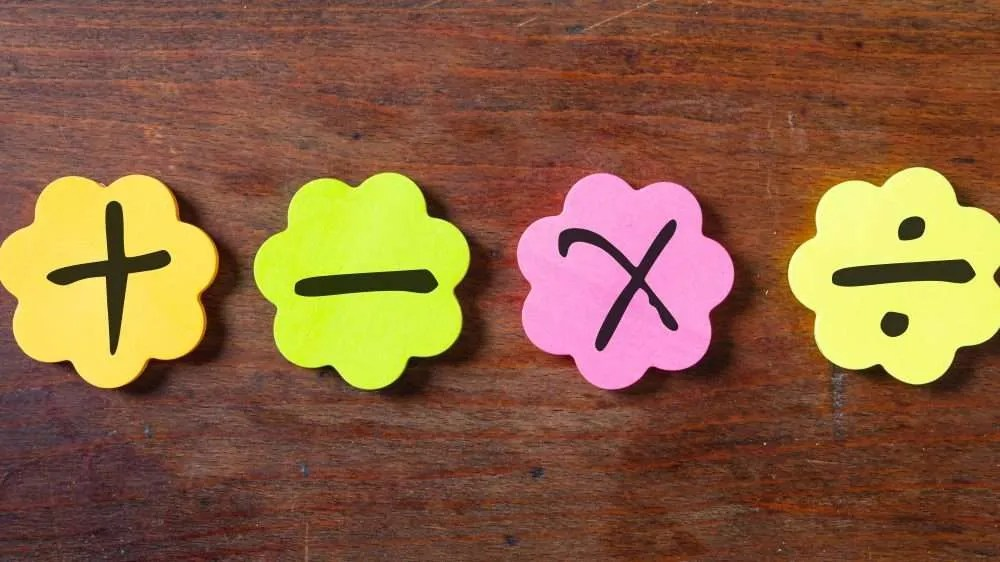Simple multiplication and division

These slides will give you some help to multiply and divide by 0, 1, 2, 5 & 10. Interactive …

, , , e1 e2 e3 self-paced-unitThese slides will help you to read and write and compare fractions. Through this unit we will look at how you can compare the relative size of different fractions as well as learning some of the terms used when talking about them. Interactive unit with …

, , , e1 e2 e3 self-paced-unit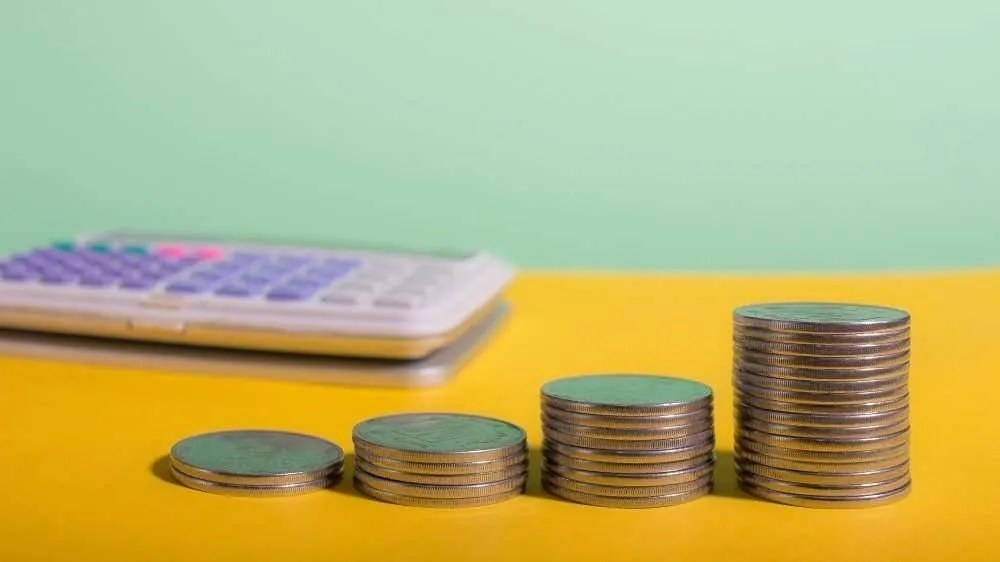Ordering decimals

In this unit we will put decimal amounts in order of size and compare decimal amounts to percentages and fractions   Interactive unit with quiz …

, , , e1 e2 e3 self-paced-unit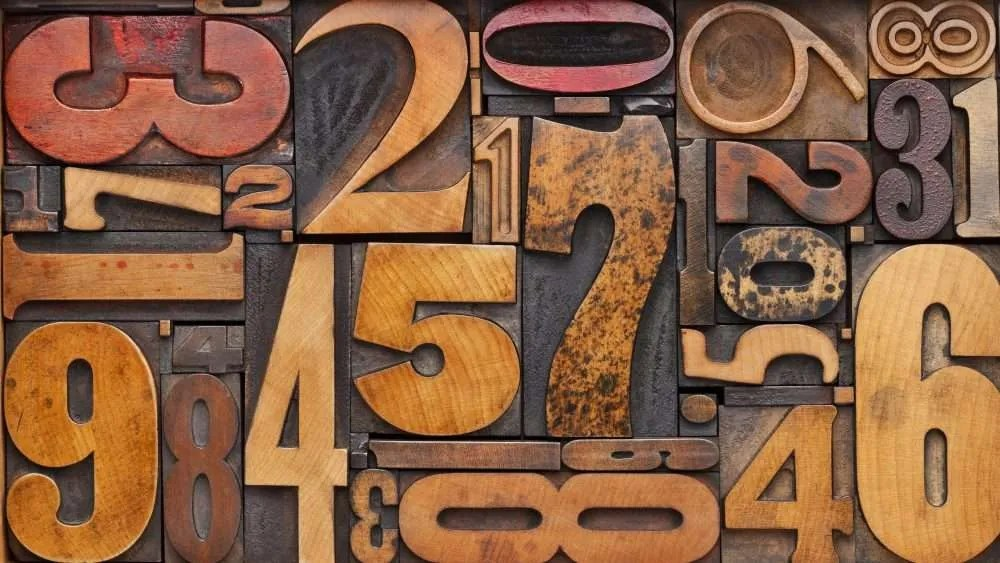In this unit we will look at strategies for working out larger calculations in addition and subtraction. This is typically known as column working. Interactive …

, , , e1 e2 e3 self-paced-unit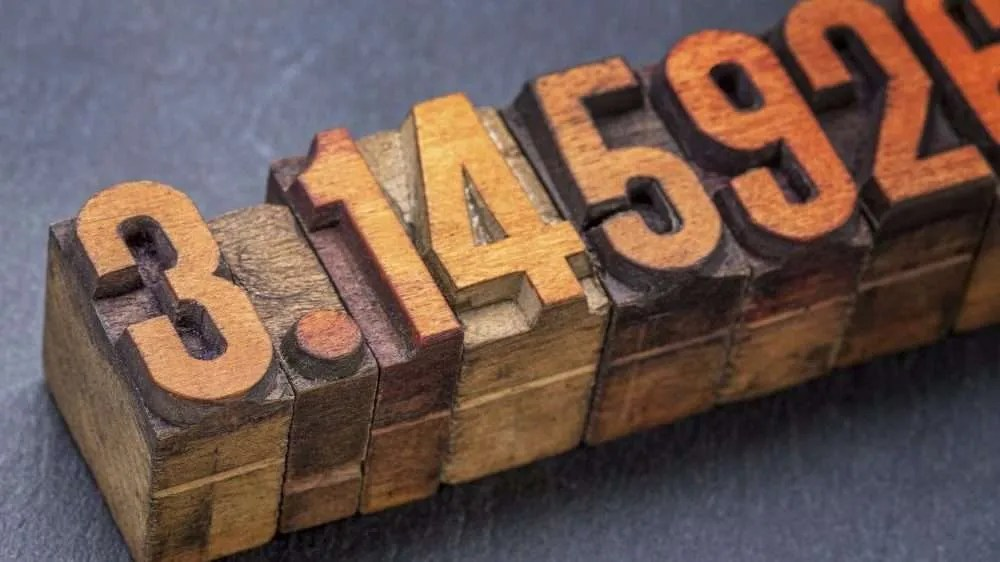Converting decimals

These slides will help you to convert decimals to fractions and percentages. This will help you to put them in order of size. Interactive unit with …

, , l1 l2 self-paced-unitAverages

In this video we look at how to work out the average of a set of numbers as well as the reasons why this can be such a useful skill. Video – 3 …

, , , , , e1 e2 e3 l1 l2 videoProbability

Probability is how we look at the chances of something happening. It’s a useful skill for all sorts of reasons and, in this video, we’ll be taking a look at how to calculate and use probability to your advantage. Video – 3 …

, , , , , e1 e2 e3 l1 l2 videoInterest

Interest is a topic that often confuses people. In this video we look at how to work out both simple and compound forms of interest. Animated video – 5 …

, , animated-video l1 l2Approximations and rounding

This module shows you how do rounding to a whole number or to one or two decimal places, followed an exercises to work through. Interactive …

, , l1 l2 self-paced-unitRatio & Proportion

Ratio and Proportion are skills that we use every day without even thinking about it. This video takes a look at where we find these skills in everyday life and how we can use them properly in exams and work. Video  – 5 …

, , , , , e1 e2 e3 l1 l2 video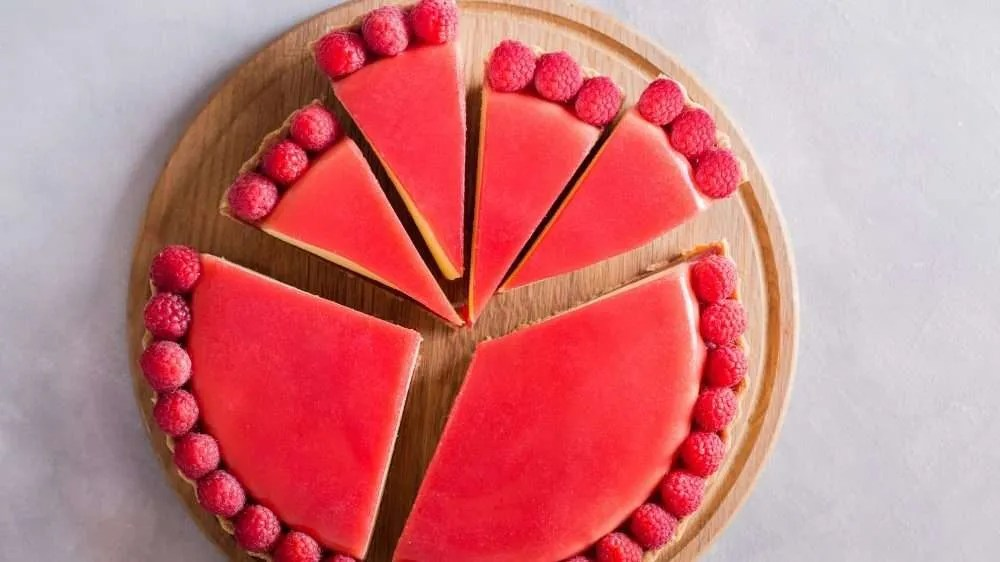Using fractions

What is a fraction? When an object is divided into a number of equal parts, each part is called a fraction. Let’s take a look… Interactive unit with …

, , l1 l2 self-paced-unitFractions, decimals and percentages

In this video we look at the relationship between fractions, decimal numbers and percentages. Video – 3:30 …

, , , , , e1 e2 e3 l1 l2 video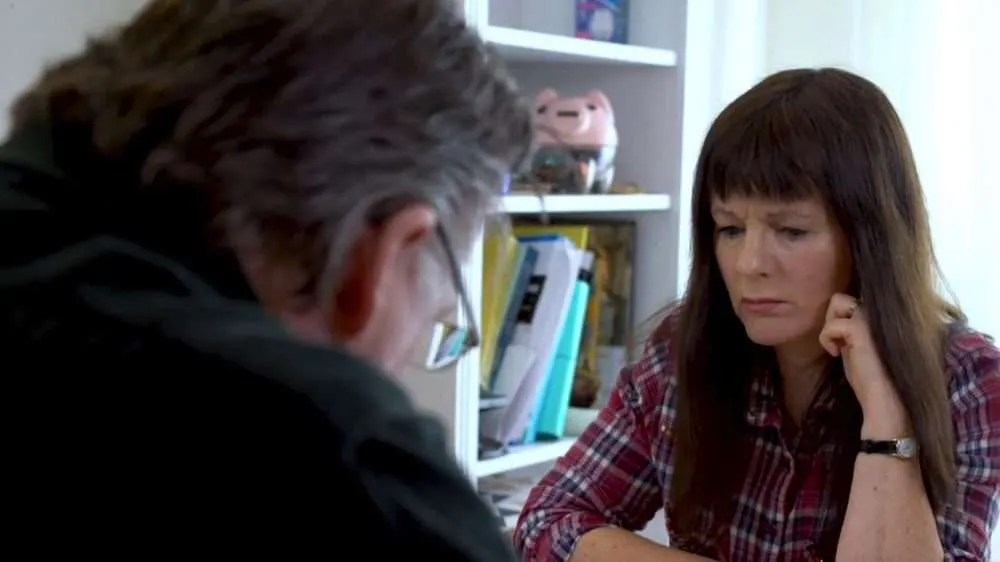Measure

In this video we look at the importance of understanding measurements. Knowing the difference between millimetres and millilitres can help you save money and get the best deals. Video – 3 …

, , , , , e1 e2 e3 l1 l2 videoEstimating numbers using rounding

This module shows you how to work out equivalent fractions followed by a number of exercises to practice. Rounding can be used as a quick way of getting a rough idea or estimate of a number. It may be a little less or a little more. There may be times when you need to provide a number that does not need to be exact. Rounding makes it easier and faster, and its near enough is still useful. Estimating through rounding – …

, , , e1 e2 e3 self-paced-unitEquivalent fractions

This module shows you how to work out equivalent fractions followed by a number of exercises to practice. Equivalent fractions are two fractions that are equal to the same amount. Interactive presentation – …

, , , e1 e2 e3 self-paced-unitNumber and counting

This unit works through a series of activities to help you understand the basics of place value and counting. The unit includes the basics of negative numbers. Interactive module : 20 minutes A. Understand place value (E3) 1 B. Understand place value (E3) 2 C. Understand place value (E3) 3 D. Rounding numbers to the nearest 10 or 100 (E3) 1 E. Rounding numbers to the nearest 10 or 100 (E3) 2 F. Changing pence into pounds (E3) G. Rounding Numbers – How Far? H. Understand place value (to seven digits) I. Recognise negative and positive numbers …

, , , e1 e2 e3 self-paced-unitProbabilities

This is a short video to explain the basic concepts of probabilities and using a probability scale to compare the odds of different events. Video: Powtoon 3 minutes   Recommended resources (off-site links) BBC Bitesize : probabilities Math is fun : How to work out probabilities – American but well spread out examples Revision Maths: Higher level up to GCSE Wikihow: Also higher …

, animated-video l1Receipt calculations

A short quiz module that covers simple addition from a shopping list followed by working out the cheapest item through division and multiplication. Interactive quiz Food shopping maths 10 minutes Recommended resources (off site links) Khan Academy – excellent free online modules – Multiplication and …

, , , e3 l1 l2 self-paced-unit
Superstore calculations

This is a short interactive quiz module designed to run through some real world calculations of numbers and quantities. Tasks also include reading from charts and diagrams. Superstore Maths quiz 10 minutes   Recommended resources (off site links) Khan Academy – excellent free online modules – Ratio and …

, , l1 l2 self-paced-unitSuperstore discounts

This is a short module that covers how to work out discounts through decimal conversions.   Introduction and quiz module 10 minutes Handout and worksheet  pdf  Publisher   Recommended resources (off site links) The excellent free Khan academy. Decimal conversions unit Online decimal calculator, useful for checking students to check their own …

, , l1 l2 self-paced-unitBODMAS

VIDEO: 3 minute digital animation Bodmas (also sometimes called Bidmas) is a core maths tool. This handy mnemonic helps you to remember the correct order to use when working through sums. Brackets, Orders (or other or indices), Division, Multiplication, Addition and …

, animated-video l1Number estimation

VIDEO : 3 minute digital animation Number estimation is a core maths skill. This technique is incredibly important for checking your work and is invaluable in completing exams and not losing easy …

, , , , , animated-video e1 e2 e3 l1 l2